# MCAT Physical : Nuclear Chemistry and Electrons

## Example Questions

← Previous 1 3 4 5 6 7

### Example Question #1 : Nuclear Chemistry And Electrons

Which of the following is not accurate regarding spontaneous fission reactions?

Possible Answers:

The products have smaller binding energies per nucleon than the original atom

The two resulting nuclei are approximately equal to each other in mass and atomic number

The total kinetic energy of the products is less than the kinetic energy of the original atom

The sum of the atomic weights of the products is less than the atomic weight of the original atom

The potential energy of the system is greater after the reaction than before

Correct answer:

The products have smaller binding energies per nucleon than the original atom

Explanation:

In fission a large atom, with low binding energy per nucleon, splits into two approximately equal smaller atoms, which are more stable due to greater binding energy per nucleon. All the other properties mentioned are accurate.

### Example Question #1 : Nuclear Chemistry And Electrons

In the operation of nuclear reactors, engineers make use of substances known as neutron poisons. These are used to help store nuclear waste and slow nuclear reactions, but are also generated naturally in nuclear chain reactions as a by-product. This natural by-product can stop the desirable chain reaction present in a nuclear reactor used for power generation.

For example, in nuclear power plants, U-235 is used as a fuel. U-235 absorbs a neutron, and subsequently generates neutrons (which power the chain reaction) and Xe-135. Xe-135 is a well-known neutron poison, and thus can impact the continued chain reaction of a nuclear power plant if it becomes over abundant during power generation.

To help account for this, engineers have developed measurements to quantify the impact of Xe-135 on nuclear operations. For instance, the time during which there is an inability to start a reactor due to the buildup of Xe-135 is referred to as the precluded start-up time. Also, the amount of time that the reactor cannot override the effects of built up Xe-135 is called poison outage time. Perhaps the most important measure that engineers have developed is the neutron absorption capacity (σ), which is measured in units of barns and is a function of microscopic cross section. Xe-135 has a neutron absorption capacity of 2.00 * 106 barns, while another common poison, Sm-149, has a neutron absorption capacity of 74,500 barns.

Xe-135 is a fission product of U-235 after it absorbs a neutron. An alternative fission reaction allows U-235 to produce Ba-141 and three neutrons. If all that is produced in this fission reaction is Ba-141, three neutrons, and one other species, which of the following could be that one other species?

Possible Answers:

Kr-93

Kr-91

Kr-92

Xe-124

Xe-134

Correct answer:

Kr-92

Explanation:

This is a tricky question, because it requires two steps. The first step is to recognize that the question specifies that U-235 absorbs a neutron via fission, so it becomes U-236. U-236 then breaks down into three neutrons, Ba-141, and Kr-92, because the total mass must not change.

Uranium has 92 protons, meaning that U-236 has 144 neutrons (236 – 92 = 144). Barium has 56 protons, meaning that Ba-141 must have 85 neutrons.

The identity of the unknown compound is given by the total protons (92) minus the protons in barium (56).

92 – 56 = 36

The unknown compound has 36 protons, meaning it must be Kr. The remaining neutrons must make up the remaining mass of the krypton isotope. There are 144 neutrons total, 85 given to barium, and 3 lost during reaction.

144 – (85 + 3) = 56

Krypton has 56 neutrons and 36 protons, giving it a total mass of 92.

### Example Question #1 : Atomic Nucleus

Which of the following is not a characteristic of nuclear fission?

Possible Answers:

The combined mass of the resulting nuclei is less than the mass of the original nucleus

Fission is caused when the electromagnetic force dominates the strong nuclear force

Fission of light elements is exothermic

Fission can release neutrons as well as new nuclei

Some isotopes of a particular element may perform spontaneous fission, while other isotopes do not

Correct answer:

Fission of light elements is exothermic

Explanation:

Fission of heavy elements is exothermic; it releases energy because the products are closer to the peak of the binding energy curve than the original nucleus was. In other words, the products are more tightly bound and have more negative binding energies.

In contrast, fission is endothermic for lighter elements because their fission products are farther from the peak of the binding energy curve (the products are less tightly bound). So, fission of lighter elements would require an energy input in order to occur, making light-element fission endothermic.

All other listed answers are true statements.

### Example Question #2 : Atomic Nucleus

Which of the following are correct statements about binding energy?

I. Light nuclei experience an increase in binding energy by fusion, and heavy nuclei by fission

II. Binding energy is the amount of energy required to separate a nucleus into its individual nucleons

III. Radioactive decay processes tend to produce nuclei which have lower binding energies than the original nucleus

Possible Answers:

I and III only

I and II only

II and III only

III only

I, II, and III

Correct answer:

I and II only

Explanation:

The first two statements are true. Statement III is false becuse radioactive decays tend to produce nuclei with higher binding energy per nucleon than the parent nucleus, since nuclei with higher binding energy are held together more tightly and thus are more stable.

### Example Question #1 : Nuclear Chemistry And Electrons

In the operation of nuclear reactors, engineers make use of substances known as neutron poisons. These are used to help store nuclear waste and slow nuclear reactions, but are also generated naturally in nuclear chain reactions as a by-product. This natural by-product can stop the desirable chain reaction present in a nuclear reactor used for power generation.

For example, in nuclear power plants, U-235 is used as a fuel. U-235 absorbs a neutron, and subsequently generates neutrons (which power the chain reaction) and Xe-135. Xe-135 is a well-known neutron poison, and thus can impact the continued chain reaction of a nuclear power plant if it becomes over abundant during power generation.

To help account for this, engineers have developed measurements to quantify the impact of Xe-135 on nuclear operations. For instance, the time during which there is an inability to start a reactor due to the buildup of Xe-135 is referred to as the precluded start-up time. Also, the amount of time that the reactor cannot override the effects of built up Xe-135 is called poison outage time. Perhaps the most important measure that engineers have developed is the neutron absorption capacity (σ), which is measured in units of barns and is a function of microscopic cross section. Xe-135 has a neutron absorption capacity of 2.00 * 106 barns, while another common poison, Sm-149, has a neutron absorption capacity of 74,500 barns.

How does a nuclear fusion reaction compare to the nuclear fission reaction described in the passage?

Possible Answers:

Fission reactions generate energy, while fusion reactions do not

Fusion reactions generate gamma radiation, while fission reactions only generate alpha radiation

Both have unstable intermediates

Fission reactions use less massive species as reactants

Fission reactions generate gamma radiation, while fusion reactions only generate alpha radiation

Correct answer:

Both have unstable intermediates

Explanation:

Both reactions have the potential to produce energy, and both reactions have unstable intermediates. Fission reactions have unstable intermediates in the form of species like Xe-135, which the passage describes as being reactive with neutrons to become a different chemical species. Similarly, fusion reactions fuse smaller nuclei into unstable larger nuclei that subsequently give off energy upon their decay.

### Example Question #1 : Nuclear Chemistry And Electrons

In the operation of nuclear reactors, engineers make use of substances known as neutron poisons. These are used to help store nuclear waste and slow nuclear reactions, but are also generated naturally in nuclear chain reactions as a by-product. This natural by-product can stop the desirable chain reaction present in a nuclear reactor used for power generation.

For example, in nuclear power plants, U-235 is used as a fuel. U-235 absorbs a neutron, and subsequently generates neutrons (which power the chain reaction) and Xe-135. Xe-135 is a well-known neutron poison, and thus can impact the continued chain reaction of a nuclear power plant if it becomes over abundant during power generation.

To help account for this, engineers have developed measurements to quantify the impact of Xe-135 on nuclear operations. For instance, the time during which there is an inability to start a reactor due to the buildup of Xe-135 is referred to as the precluded start-up time. Also, the amount of time that the reactor cannot override the effects of built up Xe-135 is called poison outage time. Perhaps the most important measure that engineers have developed is the neutron absorption capacity (σ), which is measured in units of barns and is a function of microscopic cross section. Xe-135 has a neutron absorption capacity of 2.00 * 106 barns, while another common poison, Sm-149, has a neutron absorption capacity of 74,500 barns.

In Xe-135, if each neutron and proton were separated and organized into 135 separate particles, then weighed and the masses summed precisely, the total of their masses would be equal to 135 atomic mass units.

Which of the following is true of the nucleus of Xe-135 if it were weighed on its own?

Possible Answers:

Its total mass would be exactly equal to 135amu

The total mass would be less than 135amu, but only if this lost mass could be directly transferred to another chemical species

Its total mass would be more than 135amu

The total mass would depend on conditions, and could be either more or less than 135amu

Its total mass would be less than 135amu

Correct answer:

Its total mass would be less than 135amu

Explanation:

The concept of mass deficit dictates that nuclear subatomic particles typically have lower mass in their bound state than they do individually. If you took the total mass of each unbound constituent, it would be higher than the mass of the bound nucleus. This is the case regardless of whether Xe-135 interacts with another chemical species.

### Example Question #1 : Nuclear Chemistry And Electrons

The mass of a certain nucleus is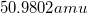. If the nucleus contains 24 protons and 27 neutrons, calculate its mass defect.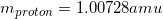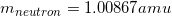Possible Answers: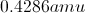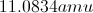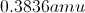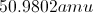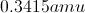Correct answer:Explanation:

When protons and neutrons combine to form the nucleus of an atom, they generally have less total energy than the energies of the individual nucleons combined. This difference is known as the mass defect. In this case, the nucleus has 24 protons, each with a mass of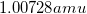, and 27 neutrons, each with a mass of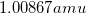.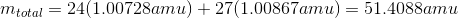The mass defect will be the difference between this predicted mass and the actual measured mass.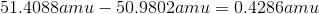### Example Question #1 : Atomic Nucleus

Which of the following cannot be the mass a nucleus containing two protons and two neutrons?

Possible Answers: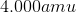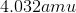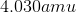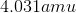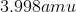Correct answer:Explanation:

The mass of a proton is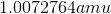and the mass of a neutron is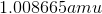. The mass of the given nucleus would be: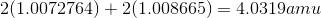Because of mass deficit, it is not possible to get a mass greater than this value. The mass of a nucleus is always less than the sum of the masses of the parts.

### Example Question #1 : Nuclear Chemistry And Electrons

In the operation of nuclear reactors, engineers make use of substances known as neutron poisons. These are used to help store nuclear waste and slow nuclear reactions, but are also generated naturally in nuclear chain reactions as a by-product. This natural by-product can stop the desirable chain reaction present in a nuclear reactor used for power generation.

For example, in nuclear power plants, U-235 is used as a fuel. U-235 absorbs a neutron, and subsequently generates neutrons (which power the chain reaction) and Xe-135. Xe-135 is a well-known neutron poison, and thus can impact the continued chain reaction of a nuclear power plant if it becomes over abundant during power generation.

To help account for this, engineers have developed measurements to quantify the impact of Xe-135 on nuclear operations. For instance, the time during which there is an inability to start a reactor due to the buildup of Xe-135 is referred to as the precluded start-up time. Also, the amount of time that the reactor cannot override the effects of built up Xe-135 is called poison outage time. Perhaps the most important measure that engineers have developed is the neutron absorption capacity (σ), which is measured in units of barns and is a function of microscopic cross section. Xe-135 has a neutron absorption capacity of 2.00 * 106 barns, while another common poison, Sm-149, has a neutron absorption capacity of 74,500 barns.

What is the force responsible for attracting neutrons to the nuclei of neutron poisons?

Possible Answers:

Electroweak force

Electrostatic force

Strong nuclear force

Gravitational force

Covalent force

Correct answer:

Strong nuclear force

Explanation:

The subatomic particles in an atom's nucleus, protons and neutrons, are held together by their own force called the strong nuclear force. Strong nuclear forces act in a somewhat counterintuitive way, and only over very short distances. The strong force is what allows protons to overcome their electrical repulsion to one another and exist together in the nucleus.

### Example Question #1 : Nuclear Chemistry And Electrons

In the nucleus, the accumulation of positive charges creates an electrostatic repulsion, known as the electromagnetic force. With the repulsive force that is generated, what is responsible for binding atoms together?

Possible Answers:

The nucleus is constantly disintegrating and reforming, giving off the appearance that it is bound together

The binding energy of the strong force is greater than the electrostatic repulsion of the electromagnetic force

The presence of neutrons are able to neutralize the repulsive force

The gravitational force on Earth binds nucleons together

Correct answer:

The binding energy of the strong force is greater than the electrostatic repulsion of the electromagnetic force

Explanation:

The binding energy of atoms is due in large part to the nuclear force, or strong force, which is the most powerful of the four universal forces.

Because the magnitude of the strong force is so large, it is able to overcome the electromagnetic force that is generated by the repulsion of like charges. Atoms exist in other parts of the universe beyond Earth, so the gravitational force is clearly not responsible. Neutrons have "neutral" charge, but that does not mean they are able to "neutralize" positive or negative charges.

← Previous 1 3 4 5 6 7

### All MCAT Physical Resources